﻿ JavaScript基础语法规则 – 码云笔记

# JavaScript基础语法规则

119℃

[隐藏]

## 什么是变量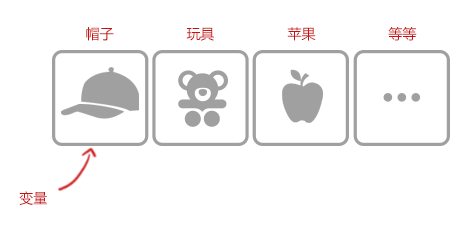## 变量命名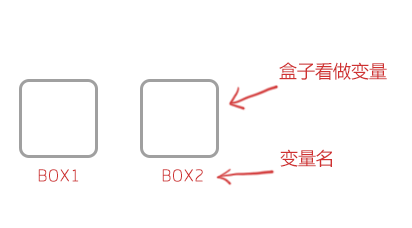1.必须以字母、下划线或美元符号开头，后面可以跟字母、下划线、美元符号和数字。如下:

``````正确:
mysum
_mychar
\$numa1``````
``````错误:
6num  //开头不能用数字
%sum //开头不能用除(_ \$)外特殊符号,如(%  + /等)
sum+num //开头中间不能使用除(_ \$)外特殊符号，如(%  + /等)``````

2.变量名区分大小写，如:A与a是两个不同变量。

3.不允许使用JavaScript关键字和保留字做变量名。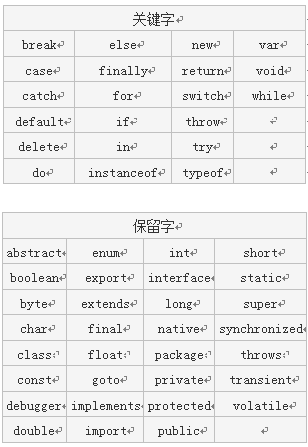## 变量声明

``声明变量语法: var 变量名; ``

var就相当于找盒子的动作，在JavaScript中是关键字（即保留字），这个关键字的作用是声明变量，并为”变量”准备位置(即内存）。

``var mynum ; //声明一个变量mynum``

``var num1,mun2 ; //声明一个变量num1``

## 变量赋值

``var mynum = 5 ; //声明变量mynum并赋值。``

``````var mynum; //声明变量mynum
mynum = 5 ; //给变量mynum赋值``````

``````var num1 = 123;       // 123是数值
var num2 = "一二三";    //"一二三"是字符串
var num3=true;    //布尔值true（真），false(假)``````

## 表达式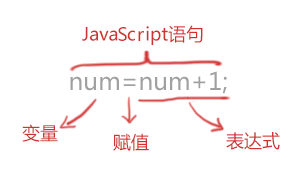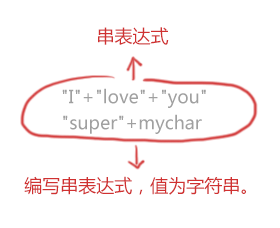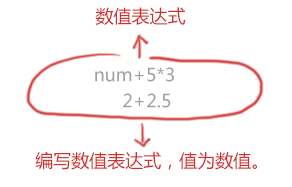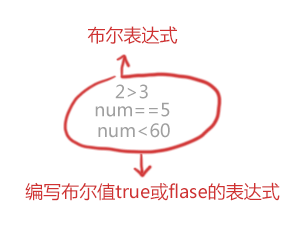## +号操作符

（1）操作符

``sum = numa + numb;``

JavaScript中还有很多这样的操作符，例如，算术操作符(+、-、*、/等)，比较操作符(<、>、>=、<=等)，逻辑操作符(&&、||、！)。

(2) “+”操作符

``mystring = "Java" + "Script"; // mystring的值“JavaScript”这个字符串``

## 自加一，自减一 ( ++和- -)

``````mynum = 10;
mynum++; //mynum的值变为11
mynum--; //mynum的值又变回10``````

``````mynum = mynum + 1;//等同于mynum++
mynum = mynum - 1;//等同于mynum--``````

## 比较操作符

``````我们先来做道数学题，数学考试成绩中，小明考了90分，小红考了95分，问谁考的分数高?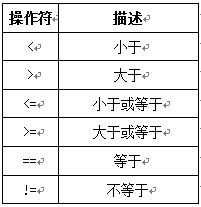``````var a = 5;//定义a变量，赋值为5
var b = 9; //定义b变量，赋值为9
document.write (a<b); //a小于b的值吗? 结果是真(true)
document.write (a>=b); //a大于或等于b的值吗? 结果是假(false)
document.write (a!=b); //a不等于b的值吗? 结果是真(true)
document.write (a==b); //a等于b的值吗? 结果是假(false)``````

## 逻辑与操作符

``b>a && b<c    //“&&”是并且的意思, 读法"b大于a"并且" b小于c "``

``````if(有准考证 &&有身份证)
{
进行考场考试
}``````

“&&”是逻辑与操作符，只有“&&”两边值同时满足(同时为真)，整个表达式值才为真。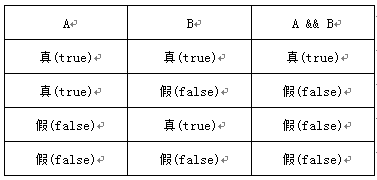## 逻辑或操作符

“||”逻辑或操作符，相当于生活中的“或者”，当两个条件中有任一个条件满足，“逻辑或”的运算结果就为“真”。

``````var a=3;
var b=5;
var c;
c=b>a ||a>b;  //b>a是true，a>b是false，c是true``````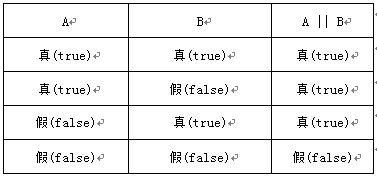## 逻辑非操作符

“!”是逻辑非操作符，也就是”不是”的意思,非真即假，非假即真。好比小华今天买了一个杯子，小明说:”杯子是白色的”，小亮说:“杯子是红色的”，小华说：”小明说的不是真话，小亮说的不是假话”。猜猜小华买的什么颜色的杯子，答案：红色杯子。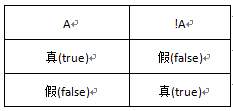``````var a=3;
var b=5;
var c;
c=!(b>a);  // b>a值是true,! (b>a）值是false
c=!(b<a);  // b<a值是false, ! (b<a）值是true``````

## 操作符优先级

``````var numa=3;
var numb=6
jq= numa + 30 / 2 - numb * 3;  // 结果为0``````

``````var numa=3;
var numb=6
jq= ((numa + 30) / (2 - numb)) * 3; //结果是-24.75``````

``````var numa=3;
var numb=6;
jq= numa + 30 >10 && numb * 3<2;  //结果为false``````